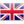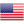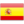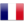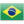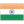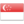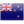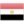» » » How to calculate the support layer spacing and support data of steel sheet pile？

How to calculate the support layer spacing and support data of steel sheet pile？

Views:5

Steel sheet pile is widely used in the harbor, river, special prevention of stone sliding or soil sand collapse, cut-off and other engineering fields, with good quality and efficiency of construction. Steel sheet pile support within the layer spacing and support is a certain scientific basis, not free to say it is a supporting algorithm of the expert below we Hengyong construction will bring everyone together to learn to analyze related technical aspects of the use of steel sheet pile by real data, we come to the specific look.

Calculation method of steel sheet pile supporting data

Data: 1 pile top elevation of H1:4.100m H2:3.000m Level 2 construction ground elevation H0:4.350m excavation bottom elevation H3:-3.400m excavation depth H:7.7500m 3 soil bulk density and soil 1:18.3KN/m3 average value of gamma gamma 'float density: 10.0KN/m3 internal friction angle and average diameter: b=9.0m long a=20.0m wide excavation excavation pit 20.10 ~ 4 were subsequently q:20.0KN/m2 5 pit

(1) the external force of steel sheet pile, the earth pressure intensity and pressure distribution ka=tg2 (45 degree - Phi /2) =tg2 (45-20.10/2) =0.49 kp=tg2 (45 DEG + phi /2) =tg2 (45+20.10/2) =2.05 on sheet pile are introduced

The load distribution is Pa1, Pa1=r x (h+0.25) Ka=18.3 x (1.09+0.25) * 0.49=12.0KN/m2 above the pile top, and the soil height is h, h=q/r=20.0/18.3=1.09m above the pile top

The water level of earth pressure strength of Pa2 Pa2=r (h+4.35 -3.00 Ka=18.3) * * * (1.09+4.35 -3.00) 0.49=21.8KN/m2 the earth pressure on the excavation face strength of Pa3 * Pa3=[r (h+4.35 + -3.00) (r-rw) (3.00+3.40)}Ka * =[18.3 (1.09+4.35 -3.00) + (18.3-10) x (3.00+3.40)] * 0.49= 47.8KN/m2 (cofferdam excavation face water pressure pump after) Pa4:Pa4= gamma (3.00+3.40) * =10 (3.00+3.40) =64.0KN/m2

Two, steel plate pile support layer spacing

The layout of steel sheet piles should be arranged according to the equal bending distance, and the maximum bending distance can be taken to determine the maximum allowable span of the cantilever end of the sheet pile According to the calculation formula of steel sheet pile, What are the functions and advantages of Larson steel sheet piles sigma =M/Wz gets concrete and accurate data. Maximum bending moment M0=Wz * (sigma]=189.0KN*m)

Contact with us

Shunli Steel Group
Tel. : 0086 -25 - 84722733
Fax: 0086 - 25 - 84730966
Hand phone: +86 13905190622